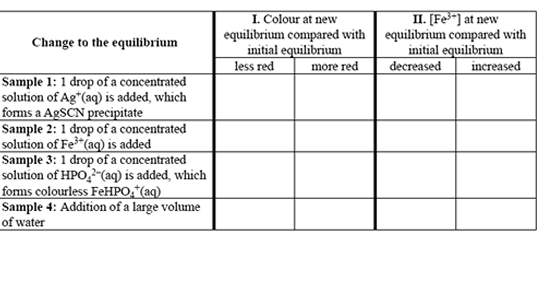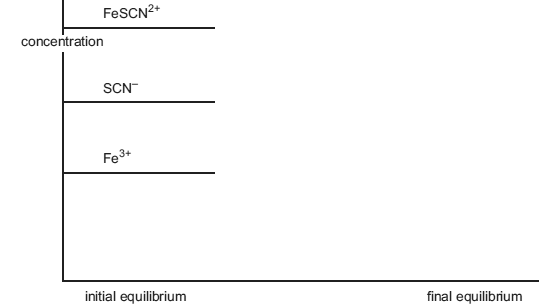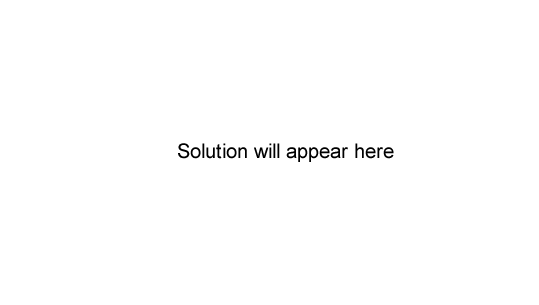Equilibrium chemistry 2006 VCE In solution, pale yellow-coloured Fe3+(aq) and colourless SCN(aq) form an equilibrium with FeSCN2+(aq). FeSCN2+(aq) is red in colour. Fe3+(aq) + SCN(aq) => FeSCN2+(aq); ΔH negative A student investigates this reaction using separate samples of an equilibrium mixture in which significant quantities of Fe3+, SCN and FeSCN2+ are present. In each case changes are made as indicated in the table on the right. Complete the table by placing ticks in the appropriate boxes to indicate the effect of each change on I. the intensity of the red colour of the solution; and II. the concentration of Fe3+(aq) once the new equilibrium has been established. SolutionThe reaction is exothermic. The graph on the right represents the initial concentrations of the ions at equilibrium. Sketch the changes that would be expected to occur to these concentrations if the temperature of the equilibrium mixture was increased to a new, constant value. SolutionGiven the following information Cl2(g) =>2Cl(g); K = 1.13 × 10-6 M at 1100°C what would be the numerical value of the equilibrium constant for the reaction. 2Cl(g) => Cl2(g) at the same temperature? A. 8.85 × 105 B. 4.42 × 105 C. 2.26 × 106 D. 1.13 × 106 SolutionTransport of oxygen in the body involves the complex molecules haemoglobin and oxyhaemoglobin. haemoglobin + oxygen <=> oxyhaemoglobin If carbon monoxide (CO) is present in the air, poisoning can occur because. A. the equilibrium constant, K, for the reaction is reduced. B. CO reacts with oxygen to form CO2, driving the equilibrium to the left. C. the equilibrium shifts to the left because haemoglobin bonds strongly with CO. D. CO catalyses the decomposition of oxyhaemoglobin into haemoglobin and oxygen. Solution